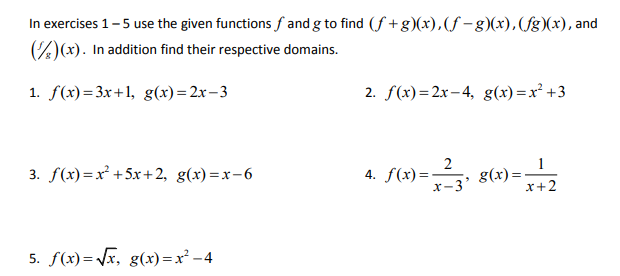# In exercises 1-5 use the given functions f and g to find (f +g)(x),(f -g)(x), (fg)(x), and )(x). In addition find their respective domains. 1. f(x)=3x+1, g(x)=2x-3 2. f(x)=2x– 4, g(x)=x² +3 3. f(x)=x² +5x+ 2, g(x) =x-6 4. f(x)= x-3' g(x)= x+2 5. f(x)= Vx, g(x) =x² -4

Question

Thankshelp_outlineImage TranscriptioncloseIn exercises 1-5 use the given functions f and g to find (f +g)(x),(f -g)(x), (fg)(x), and )(x). In addition find their respective domains. 1. f(x)=3x+1, g(x)=2x-3 2. f(x)=2x– 4, g(x)=x² +3 3. f(x)=x² +5x+ 2, g(x) =x-6 4. f(x)= x-3' g(x)= x+2 5. f(x)= Vx, g(x) =x² -4 fullscreen

### Want to see this answer and more?

Experts are waiting 24/7 to provide step-by-step solutions in as fast as 30 minutes!*

*Response times may vary by subject and question complexity. Median response time is 34 minutes for paid subscribers and may be longer for promotional offers.
Tagged in
Math
Algebra

### Other